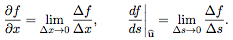18.02SC | Fall 2010 | Undergraduate

# Multivariable Calculus

## 2. Partial Derivatives

« Previous | Next »

In this unit we will learn about derivatives of functions of several variables. Conceptually these derivatives are similar to those for functions of a single variable.

1. They measure rates of change.
2. They are used in approximation formulas.
3. They help identify local maxima and minima.

As you learn about partial derivatives you should keep the first point, that all derivatives measure rates of change, firmly in mind. Said differently, derivatives are limits of ratios. For example,Of course, we’ll explain what the pieces of each of these ratios represent.

Although conceptually similar to derivatives of a single variable, the uses, rules and equations for multivariable derivatives can be more complicated. To help us understand and organize everything our two main tools will be the tangent approximation formula and the gradient vector.

Our main application in this unit will be solving optimization problems, that is, solving problems about finding maxima and minima. We will do this in both unconstrained and constrained settings.

Part A: Functions of Two Variables, Tangent Approximation and Optimization

Part B: Chain Rule, Gradient and Directional Derivatives

Part C: Lagrange Multipliers and Constrained Differentials

Exam 2

« Previous | Next »

## Course Info

Fall 2010
##### Learning Resource Types
Simulations
Exams with Solutions
Problem Sets with Solutions
Lecture Videos
Lecture Notes